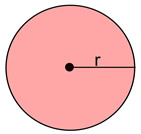Visualizing Polynomials Terms of Use    Contact Person: Donna RobertsDirections: Answer these questions pertaining to polynomials. Check each answer when finished.

1.
Find the perimeter of the regular pentagon shown at the right.

Choose:
 5x2 + 2x + 6 5x2 + 10x + 30 5x2 + 10x + 12 6x2 + 12x + 36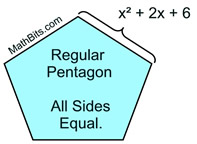2.
The diagram at the right can be used to visualize the value of (x + 6)2. Which choice is NOT supported by the diagram?

Choose:
 (x + 6)2 = x2 + 4[3(x + 3)] (x + 6)2 = x2 + 2•3x + 2•3(x + 6) (x + 6)2 = x2 + 4[(x + 6)•3] (x + 6)2 = x2 + 4•3x + 4•9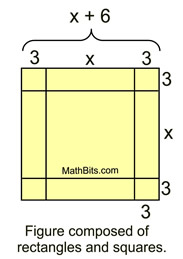3.
The diagram at the right is composed of a square and two rectangles. Find a polynomial expression for the total area of the figure in square units.

Choose:
 x2 + 7x x2 + 10x x2 + 7x + 10 x2 + 6x + 54.
The diagram at the right is composed of two rectangles. Find a polynomials expression for the area of the shaded (green) region in square units.

Choose:
 19x + 25 19x + 23 29x + 25 29x + 23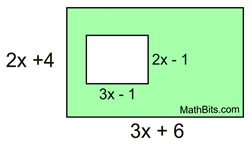Figure composed of two rectangles.

5.
How many times greater is the area of the figure on the right than the figure on the left?

Choose:
 12 times 2 times 3 times 6 times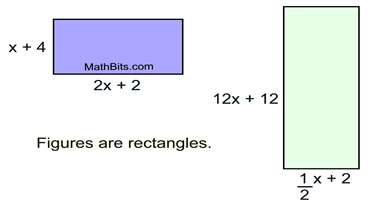6.
Express the volume of the rectangular solid seen at the right, in cubic units.

Choose:
 2x2 - x - 15 2x3 - 11x2 - 15x 2x3 + 11x2 + 15 2x3 - x2 - 15x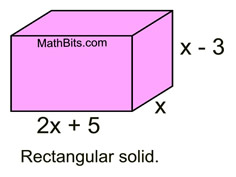7.
The volume of the box at the right is
2m3 + 5m2 +3m cubic units.
Which choice represents d in terms of m?

Choose:
 m - 1 m + 1 2m + 1 2m - 1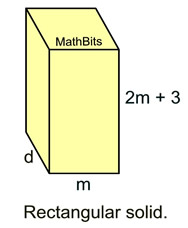8.
The area of a circle formula is A = πr2, where r is the radius of the circle. The area of the circle at the right is given as 4πx2 - 20πx + 25π. Express the radius, r, of the circle, in terms of x.

Choose:
 x - 5 2x + 5 x + 5 2x - 5